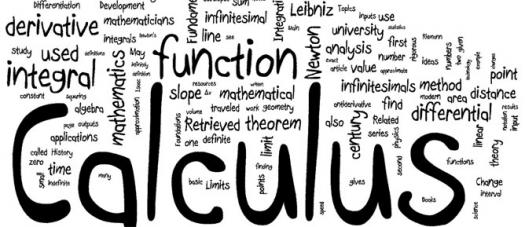# Analyzing Functions With Calculus Assessment Test

10 Questions | Total Attempts: 102SettingsWe analyze functions with the use of calculus to tell us where a function increases or decreases, concave up or down, where it has inflection points and maximum or minimum points.

• 1.
At an inflection point, a function switches from being a convex function to being a...
• A.

Concave function

• B.

Low function

• C.

Curve

• D.

Tangent

• 2.
Newton's notation for differentiation is also called the...
• A.

Label

• B.

Dot notation

• C.

Mark

• D.

Slope

• 3.
The Euler's notation is denoted by the letter...
• A.

A

• B.

B

• C.

C

• D.

D

• 4.
The gradient determines a vector field.
• A.

True

• B.

False

• C.

Sometimes

• D.

Depends on some factors

• 5.
All continuous functions and many other functions can be differentiated using...
• A.

Tangent

• B.

Weak derivative

• C.

Strong derivative

• D.

Drive

• 6.
The discrete equivalent of differentiation is...
• A.

Finite differences

• B.

Infinite differences

• C.

Difference

• D.

Derivatives

• 7.
A fundamental statement in number theory that asserts that there are infinitely many prime numbers is...
• A.

Lib Law

• B.

Theorem

• C.

Theory

• D.

Euclid's theorem

• 8.
Every integer has a unique prime factorization.
• A.

True

• B.

False

• C.

Maybe

• D.

Depends on some factors

• 9.
The action of computing a derivative is termed...
• A.

Arrival

• B.

Derivation

• C.

Differentiation

• D.

Graph

• 10.
The reverse process of differentiation is...
• A.

Deceleration

• B.

Integration

• C.

Division

• D.

Subtraction

Related TopicsBack to top